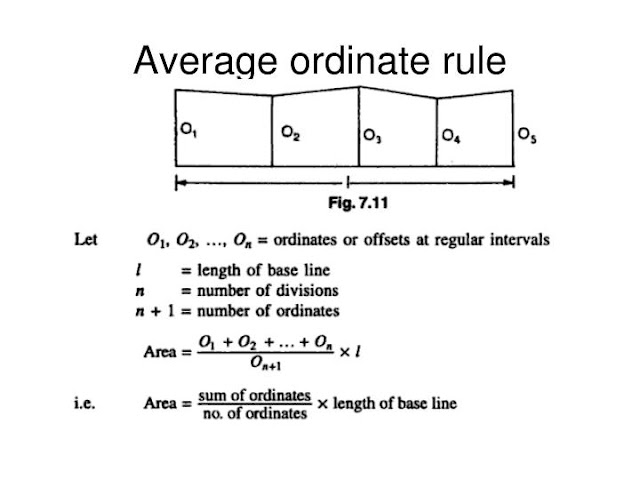# DIFFERENT METHODS OF AREA CALCULATION IN SURVEYING

In this article we will discuss DIFFERENT METHODS OF AREA CALCULATION IN SURVEYING. Using Mid-Ordinate Rule, The Average Ordinate Rule, The Trapezoidal Rule, Simpson’s Rule.

### The Mid-Ordinate Rule:

In this method, the ordinates are measured at the mid points of each division and the area is calculated by the formula;

Area = [ h1 + h2 + h3 + h4+ h5 + . . . . . . . . + hn / n] x L
OR
Area = (h1 + h2 + h3 + h4+ h5 + . . . . . + hn) x d
Where;
h1, h2, …. = The ordinates of the mid points of each division
L = The length of the base line
n = The number of equal parts into which the base line is divided.
d = The common distance between the ordinates = L/n

### The Average Ordinate Rule:

In this method, the ordinates are drawn and scaled at each of the points of division of the base line. The average of these ordinates multiplied by the length of the base line gives the required area.Add caption

Area = [ O0 + O1 + O2 + O3+ O4 + . . . . . . . . + On / n+1] x L
Where;
O0, O1, …. = The ordinates at each of the point division
L = The length of the base line
n = The number of equal parts into which the base line is divided.

### The Trapezoidal Rule:

This rule is more accurate than the first two ones. In this method, the area is divided into a series of trapezoids. The rule may be stated as:
“To the sum of the first and last ordinates, add twice the some of the intermediate ordinates. Multiply the total sum thus obtained by the common distance between the ordinates. One half of this product gives the required area”.

# TOP 20 MCQS IN ROAD CONSTRUCTION

Area = [ (O0 + On / 2) + O2+ O3 . . . . . . . . + On-1]

### Simpson’s Rule: It may be stated as: “To the sum of the first and last ordinates, add twice the sum of the remaining odd ordinates and four times the sum of all the even ordinates. Multiply the total sum thus obtained by one-third of the common distance between the ordinates and the result gives the required area”.

Area = d/3[ (OO + 4O1 + 2O2+ 4O3 + 2O4 + . . . . . . . . + 2On-2 + 4On-1+ On)]